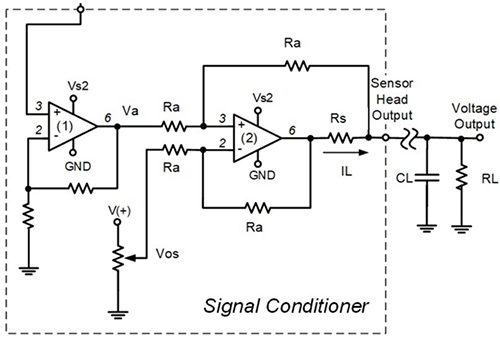High investment rates, which are usually triggered by sensory costs, are one of the obstacles to condition monitoring applications. To reduce this obstacle, the use of cost-effective sensors for such applications is required. A Micro-Electro Mechanical Systems (MEMS) accelerometer is one of the available options.

MEMS accelerometers are divided into two main categories: piezoelectric and capacitive accelerometers. Piezoresistive accelerometers consist of a one-degree-of-freedom system of a mass suspended by a spring. The MEMS accelerometer also has a cantilever beam with proof mass at the end of the beam and a piezoresistive patch on the beam core. The mass inertia causes the size difference and mass of the silicon wafer material to change when the device is in motion. The electrical signal generated by the piezoresistive patch by vibration is proportional to the acceleration of the vibrating object.

The capacitance MEMS accelerometer measures the variation of the capaciance between proof of mass and a fixed conductive electrode separated by a small gap.

#### Selection of MEMS accelerometer

The choice of accelerometers depends upon several factors such as:

• Sensitivity: It is the relationship between mechanical input and electrical output. The output is typically expressed in voltage per acceleration unit.
• Amplitude range: It specifies the range of maximum acceleration that can be measured by an accelerometer.
• Amplitude Limit: It defines the highest acceleration order that can be measured by an accelerometer.
• Shock Limit: It is the maximum acceleration the accelerometer can resist without inflicting harm to the unit.
• Natural Frequency: It is the frequency at which an independent system will oscillate with a temporal shift from its rest position by one degree of freedom. This determines the usable vibration measurement range.
• Resolution: It is the smallest change in acceleration for which a change in the electrical output is noticeable.
• Amplitude linearity: It is the degree to which the resulting accelerometer will signal voltage as it transitions from excitation with the smallest to the highest level of acceleration that can be detected. This accuracy is qualified by its linearity with a desirable deviation of 1%.
• Frequency Range: The frequency range is the range of operating frequencies. The range may be limited by the electrical or mechanical properties of the transducer or the associated auxiliary device.
• Phase Shift: It is the delay time between the mechanical input and the corresponding electric output signal for hardware.

#### Condition Monitoring of Electric Motors

The block diagram of a measurement system is shown below. The main components of the sensor head are the regulator, the 3-axis capacitive MEMS accelerometer (IIS2DH), and the signal conditioner. The station is equipped with a battery, regulator, filter, and signal conditioner, as shown in Figure 1. Filtered data is sent to the data collection unit that connects to the computer via the USB interface. Sensor head is mounted on the motor such that the measuring axis is in parallel with the radial axis of the motor.##### Figure 1: Conditional Monitoring System##### Figure 2: Signal Conditioner for the output voltage of accelerometer

The physical distance between the motors and monitoring stations could be several meters, therefore the noise waves must be minimised. The voltage drop due to the cable resistance can be solved using the current loop technique. Therefore, the measuring voltage can be transferred over a long distance. As shown in Fig.2, The signal conditioner consists of an amplifier unit and a grounded voltage-to current converter. A differential amplifier is adapted to convert the output voltage from the non-inverting amplifier (Va) to current. Thus, a span-resistor (Rs) can be determined by equation (1). The offset voltage (Vos) required in this circuit can be added at the inverting pin of the second op-amp and is calculated by equation (2).

RS = Vamax-Vamin/ILmax+ILmin....................1

Vos = Va - RsIL....................2

The value of a loaded resistor, RL, can affect the output current. Too high RL can reduce the circuit’s output current due to the op-amp’s power supply limitation. Therefore, the maximum loaded resistor (RLmax) must be estimated. RLmax is calculated by equation (3).

RLmax = VS2-RSILmax/ILmax....................3

Vs2 is an op-amp supply voltage, which is estimated at 90% of the full-scale supply voltage. RL is used to convert the current back to voltage.

The increase in voltage value over time from the sensor head represents greater vibration indicating a bearing degradation over time.

Capacitive MEMS sensors can be used with a microcontroller suitable for advanced signal processing for vibration time analysis and frequency timing for predictive maintenance. Their small form factor and low power consumption can allow an independent intelligent node for remote applications.

#### Condition monitoring Kit

The STEVAL-BFA001V1B is an industrial reference design kit for condition monitoring and predictive maintenance, in a layout that is designed to meet IEC61000-4-2/4 and EN60947 requirements for industrial applications. The STSW-BFA001V2 firmware includes dedicated algorithms for advanced time and frequency domain signal processing and analysis of the high bandwidth 3D digital accelerometer for vibration monitoring. The package includes pressure, relative humidity and temperature sensor monitoring samples as well as audio algorithms for acoustic emission.

#### Stay informed

Keep up to date on the latest information and exclusive offers!